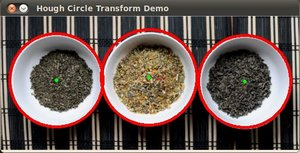# Hough Circle Transform¶

## Goal¶

In this tutorial you will learn how to:

• Use the OpenCV function HoughCircles to detect circles in an image.

## Theory¶

### Hough Circle Transform¶

• The Hough Circle Transform works in a roughly analogous way to the Hough Line Transform explained in the previous tutorial.

• In the line detection case, a line was defined by two parameters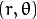. In the circle case, we need three parameters to define a circle: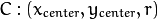where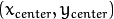define the center position (gree point) and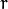is the radius, which allows us to completely define a circle, as it can be seen below: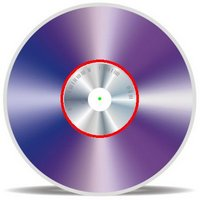• For sake of efficiency, OpenCV implements a detection method slightly trickier than the standard Hough Transform: The Hough gradient method. For more details, please check the book Learning OpenCV or your favorite Computer Vision bibliography

## Code¶

1. What does this program do?
• Loads an image and blur it to reduce the noise
• Applies the Hough Circle Transform to the blurred image .
• Display the detected circle in a window.
2. The sample code that we will explain can be downloaded from here. A slightly fancier version (which shows both Hough standard and probabilistic with trackbars for changing the threshold values) can be found here.
#include "opencv2/highgui/highgui.hpp"
#include "opencv2/imgproc/imgproc.hpp"
#include <iostream>
#include <stdio.h>

using namespace cv;

/** @function main */
int main(int argc, char** argv)
{
Mat src, src_gray;

/// Read the image
src = imread( argv, 1 );

if( !src.data )
{ return -1; }

/// Convert it to gray
cvtColor( src, src_gray, CV_BGR2GRAY );

/// Reduce the noise so we avoid false circle detection
GaussianBlur( src_gray, src_gray, Size(9, 9), 2, 2 );

vector<Vec3f> circles;

/// Apply the Hough Transform to find the circles
HoughCircles( src_gray, circles, CV_HOUGH_GRADIENT, 1, src_gray.rows/8, 200, 100, 0, 0 );

/// Draw the circles detected
for( size_t i = 0; i < circles.size(); i++ )
{
Point center(cvRound(circles[i]), cvRound(circles[i]));
int radius = cvRound(circles[i]);
// circle center
circle( src, center, 3, Scalar(0,255,0), -1, 8, 0 );
// circle outline
circle( src, center, radius, Scalar(0,0,255), 3, 8, 0 );
}

/// Show your results
namedWindow( "Hough Circle Transform Demo", CV_WINDOW_AUTOSIZE );
imshow( "Hough Circle Transform Demo", src );

waitKey(0);
return 0;
}


## Explanation¶

1. Load an image

src = imread( argv, 1 );

if( !src.data )
{ return -1; }

2. Convert it to grayscale:

cvtColor( src, src_gray, CV_BGR2GRAY );

3. Apply a Gaussian blur to reduce noise and avoid false circle detection:

GaussianBlur( src_gray, src_gray, Size(9, 9), 2, 2 );

4. Proceed to apply Hough Circle Transform:

vector<Vec3f> circles;

HoughCircles( src_gray, circles, CV_HOUGH_GRADIENT, 1, src_gray.rows/8, 200, 100, 0, 0 );


with the arguments:

• src_gray: Input image (grayscale)
• circles: A vector that stores sets of 3 values: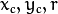for each detected circle.
• CV_HOUGH_GRADIENT: Define the detection method. Currently this is the only one available in OpenCV
• dp = 1: The inverse ratio of resolution
• min_dist = src_gray.rows/8: Minimum distance between detected centers
• param_1 = 200: Upper threshold for the internal Canny edge detector
• param_2 = 100*: Threshold for center detection.
• min_radius = 0: Minimum radio to be detected. If unknown, put zero as default.
• max_radius = 0: Maximum radius to be detected. If unknown, put zero as default
5. Draw the detected circles:

for( size_t i = 0; i < circles.size(); i++ )
{
Point center(cvRound(circles[i]), cvRound(circles[i]));
int radius = cvRound(circles[i]);
// circle center
circle( src, center, 3, Scalar(0,255,0), -1, 8, 0 );
// circle outline
circle( src, center, radius, Scalar(0,0,255), 3, 8, 0 );
}


You can see that we will draw the circle(s) on red and the center(s) with a small green dot

6. Display the detected circle(s):

namedWindow( "Hough Circle Transform Demo", CV_WINDOW_AUTOSIZE );
imshow( "Hough Circle Transform Demo", src );

7. Wait for the user to exit the program

waitKey(0);


## Result¶

The result of running the code above with a test image is shown below: